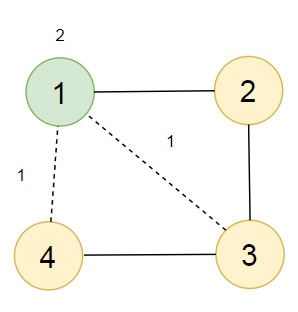# Program to find out the minimal cost so that the citizens have access to a market in Python

So, if the input is like n = 4, m = 3, x = 1, y = 2, cities = [[1, 2], [2, 3], [3, 4]], then the output will be 4.Here, we can see four cities 1, 2, 3 and 4. If a market is built a t city 1, and two more roads are built between (1, 4) and (1,3) the total cost will be 2 + 1 + 1 = 4. This is the minimum cost.

To solve this, we will follow these steps −

• if x <= y, then
• return n * x
• otherwise,
• adj_list := a map containing lists as elements
• for each city in cities, do
• city1 := city
• city2 := city
• insert city2 at the end of adj_list[city1]
• insert city1 at the end of adj_list[city2]
• temp := a new list of size (n + 1) initialized with value True
• value := 0
• dq := a double ended queue
• for cur in range 1 to n + 1, do
• if temp[cur] is non-zero, then
• value := value + x
• insert cur at the rightmost end of dq
• temp[cur] := False
• while dq is not empty, do
• for each i adj_list[extracted leftmost element of dq], do
• if temp[i] is non-zero, then
• insert i at the rightmost end of dq
• temp[i] := False
• value := value + y
• return value

## Example

Let us see the following implementation to get better understanding −

from collections import defaultdict, deque
def solve(n, m, x, y, cities):
if x <= y:
return n * x
else:
for city in cities:
city1 = city
city2 = city
temp = [True] * (n + 1)
value = 0
dq = deque()
for cur in range(1, n + 1):
if temp[cur]:
value += x
dq.append(cur)
temp[cur] = False
while dq:
if temp[i]:
dq.append(i)
temp[i] = False
value += y
return value

print(solve(4, 3, 1, 2, [[1, 2], [2, 3], [3, 4]]))

## Input

4, 3, 2, 1, [[1, 2], [2, 3], [3, 4]]


## Output

4